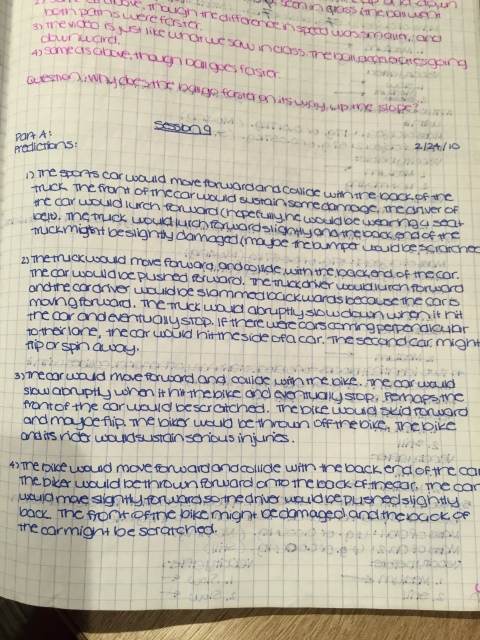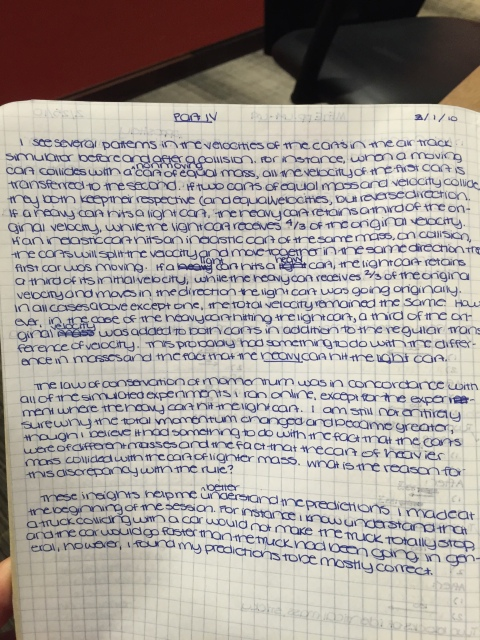# Crashes and Collisions

46 teachers like this lesson
Print Lesson

## Objective

SWBAT use a model to explain conservation of momentum and predict what happens in a collision.

#### Big Idea

In this lesson, students explore what happens when two objects collide. In particular, they examine what happens to each object after the collision.

## Getting Started

In this lesson students explore what happens when two objects collide. In particular, they will examine what happens to each object after the collision.

Goals for the lesson:

• Understand that momentum is the "oomph" of a moving object and is equal to the object's mass times its velocity (momentum = mv).
• Apply the concept of "conservation of momentum" to predict what will happen in a collision.

Materials:

• Several balls:
• Two balls of identical mass (e.g., two tennis balls)
• Two balls of unequal mass (e.g., tennis ball and styrofoam ball)
• Two yardsticks
• Masking tape
• Scale
• Two wooden blocks (1.5” sq)
• Velcro
• Laptops with internet

## Engage

5 minutes

To engage students' thinking, have them consider the following scenarios:

1.You are driving your car at 20 mph. A truck right ahead of you suddenly stops and you crash into it before you have a chance to step on the brakes.

2. A truck is moving at 20 mph. Your car is standing still at a light and the truck crashes into you before the driver has a chance to step on the brakes.

3. You are driving your car at 20 mph. A bicycle right ahead of you suddenly stops and you crash into it before you have a chance to step on the brakes.

4.  A bicycle is moving at 20 mph. Your car is stopped for a light and the bicycle crashes into you.

In your journal: Predict what you think will happen in each of these cases. Are there any tentative generalizations you might make based on these situations?

Give students time to think and write, then ask a few students to share their ideas. If you have individual white boards, this is an opportunity to use them. Have them focus their predictions on the vehicles only, not the operators.## Explore

15 minutes

Students begin by investigating collisions using balls and blocks.

1. Set up two yardsticks parallel to one another to make a track for the balls. The ball should move smoothly between the yardsticks in a straight line.

2. Students need two relatively heavy balls of medium weight, one lighter ball, two blocks, and Velcro.

3. Before they begin, weigh each object using a balance or scale.

NOTE: The phenomena they are studying is governed by rules that assume that there is no friction. Since students are working with situations in which there is some friction, precise quantitative relations will not hold, but they'll be able to see patterns in their results if they keep this in mind. Rather than keeping track of exact velocities in the experiments they conduct, keep track of direction and relative velocity in each case (e.g., slow, medium, fast). They'll have an opportunity to experiment with a simulation of a frictionless world later in the lesson.

For each of the following scenarios students will:

1. Predict how they think the objects will be moving after the collision—both their direction and relative velocity.

2. Act out the collision. Compare what happens to their prediction.

3. Watch each of the videos. How do the videos compare with their predictions and their observations?

4. Answer this question qualitatively, Because friction is affecting your experiments, quantitative measurements will not accurately reflect the physics that is occurring."

To keep track of mass and velocity before and after a collision, students will want to organize their work into a simple chart. See the Crashes and Collisions Student Handout for an idea of this.

The scenarios:

1. Two balls of equal mass — one stationary, the other collides with it.Equal Mass Video

2. Two balls of different masses—heavy ball stationary, light ball collides with it.Heavy hits light (fast)  Heavy hits light (slow)

3. Two balls of different masses—light ball stationary, heavy ball collides with it. light hits heavy

4. Two identical blocks—one block stationary, other block collides with it.Blocks collide

5. Two identical blocks—attach the opposite sides of the Velcro on each block and push one block into the other so they stick. Sticky blocks collide

Have students pull their thinking together by writing to the following prompt:

What patterns do they see in your chart? Look in particular at the relationship between the two masses. How does this relationship affect the result of the collision? Record your results in your journal.## Elaborate

20 minutes

To explore crashes and collisions in a frictionless environment use the Collision Lab from PhET, which simulates the collisions with balls.

In the simulation, students can change the mass and velocity of each object individually. They can also change the "elasticity" which controls how "bouncy" the collision is. If the blocks bounce off one another perfectly (like the ball experiments), the collision is elastic (e=1). If they stick together, like the two blocks with Velcro, the collision is inelastic (e=0).

First, students explore the simulation, trying out different combinations of masses, velocities, and values of "e." Then they use the simulation to study the collisions investigated earlier in this session:

• Two balls of identical mass
• Two balls of different mass
• Two identical blocks that stick/do not stick together.

For each situation they should draw a diagram indicating the momentum of each ball or block before and after the collision. Then students run the simulation and observe what happens.

What similarities and differences do you notice between your experiences with the balls and blocks and the simulation?## Explain

15 minutes

What happens in the situations above has to do with momentum and the conservation of momentum. We use the word momentum informally to mean how much "oomph" a person or object has; how much impetus.

In physics, momentum has a very specific definition — it is an object's mass times its velocity, or momentum = mv. Momentum has traditionally been labeled by the letter "p", so the formula as physicists write it is p = mv. This is another equation like F = ma, which relates a quantity describing motion to an object's mass, but this time it is related to the object's velocity rather than its acceleration. In our study of force, we established that if an object is not accelerating, there is no net force involved. In that case, both F and ma are 0 (zero), no matter how fast an object is moving.

But there is something very different about an object when it is moving with a very large constant velocity and when it is moving at a smaller constant velocity. One of the differences is this quantity momentum, which reflects both an object's mass and its velocity.

Momentum can be represented by a vector, just like velocity. When an object has momentum, it has momentum in a direction, the direction of its velocity. Momentum can be indicated in the same way velocity and acceleration are, as an arrow to indicate its direction and a size attached to that arrow either by length or by an explicit number. For example, a momentum of 3 mg x 5 cm/frame would be represented this way:One of the most important things about momentum is that it is conserved in collisions. That is, the amount of momentum in a system is the same before and after the collision.

To summarize their learning, have students reflect on their learning to explain the following:

1. What patterns do you see in the chart of velocities before and after a collision from your experiments with collisions? Look in particular at the relationship between the two masses. How does this relationship affect the result of the collision?
2.  How does the law of conservation of momentum help you make sense of your observations of the simulation of the balls in the PhET Collisions Lab?
3. Work with your group to come up with an understanding of conservation of momentum based on your experiences and your reading. How does this understanding apply to the situations you imagined at the beginning of the lesson?In the video below I briefly address how this lesson aligns with NGSS Science Practice 6: Constructing Explanation.